# Real Life Object Detection using OpenCV – Detecting objects in Live Video

Published  April 1, 2019   0
M
Author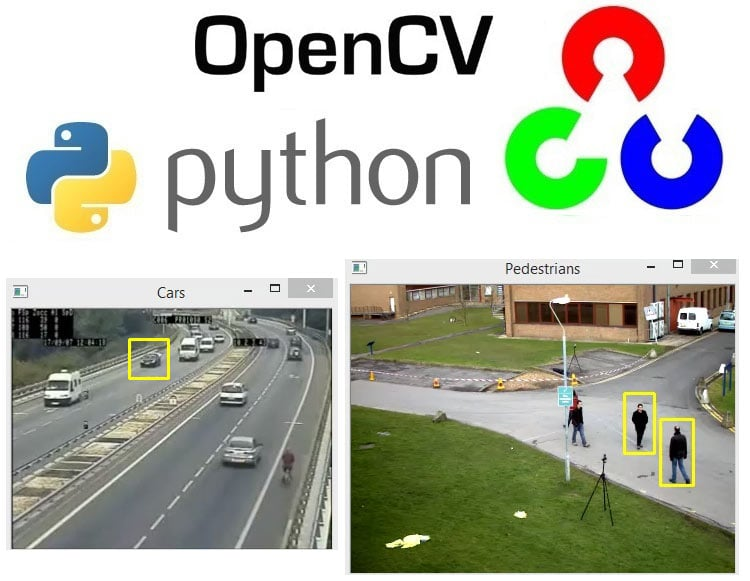We started with installing python OpenCV on windows and so far done some basic image processing, image segmentation and object detection using Python, which are covered in below tutorials:

We also learnt about various methods and algorithms for Object Detection where the some key points were identified for every object using different algorithms. In this tutorial we are going to use those algorithms to detect real life objects, here we would be using SIFT and ORB for the detection.

### Object detection using SIFT

Here object detection will be done using live webcam stream, so if it recognizes the object it would mention objet found. In the code the main part is played by the function which is called as SIFT detector, most of the processing is done by this function.

And in the other half of the code, we are starting with opening the webcam stream, then load the image template, i.e. the reference image, that is the programme is actually looking through the webcam stream.

Next, we are continuously capturing the images from the webcam stream with the help of infinite while loop, and then capturing the corresponding height and width of the webcam frame, and after then define the parameters of the region of interest (ROI) box in which our object can fit in by taking the corresponding height and width of the webcam frame. And then we draw the rectangle from the ROI parameters that we had defined above. Then finally crop the rectangle out and feed it into the SWIFT detector part of the code.

Now the SIFT detector basically have two inputs, one is the cropped image and the other is the image template that we previously defined and then it gives us some matches, so matches are basically the number of objects or keypoints which are similar in the cropped image and the target image. Then we define a threshold value for the matches, if the matches value is greater than the threshold, we put image found on our screen with green color of ROI rectangle.

Now let’s move back to the main part of the code, the function which is called as SIFT detector, it takes the input as two images one is the image where it is looking for the object and other is the object which we are trying to match to (image template). Then gray scale the first image and define the image template as second image. Then we create a SIFT detector object and run the OpenCV SIFT detect and compute function, so as to detect the keypoints and compute the descriptors, descriptors are basically the vectors which stores the information about the keypoints, and it’s really important as we do the matching between the descriptors of the images.

And then define the FLANN based matcher, we are not going into the mathematical theory of matching behind it, but you can easily Google about it. Firstly, define the index kdtree to zero and then we set the index and search parameters in the dictionary format, we just define the algorithm we are going to use which is KDTREE, and the number of trees we are going to use, the more tree we use the more complicated it gets and slower. And in search parameter define the number of checks, which is basically number of matches it’s going to complete.

And then create our FLANN based matcher object by loading the parameter we previously defined which are index parameters and search parameters and based upon this create our FLANN based matcher, which is a KNN matcher where KNN is K-nearest neighbors, basically it’s a way where we look for nearest matchers and descriptors and we do the matching with initialization constant k. Now this FLANN based matcher returns the number of matches we get.

FLANN based matching is just an approximation, so as to increase the accuracy of the FLANN based matcher we perform a Lowe’s ratio test and what it does is it looks for the matches from the knn flann based matcher and define some matric parameters which is distance here, for which distance is a numpy function, and once it meets the criteria append the matches to the good matches and returns the good matches found, and so the live video stream tells the number of matches found at the corner of the screen.

Now Let’s look at the code for the above description:

```import cv2
import numpy as np

def sift_detector(new_image, image_template):
# Function that compares input image to template
# It then returns the number of SIFT matches between them
image1 = cv2.cvtColor(new_image, cv2.COLOR_BGR2GRAY)
image2 = image_template

# Create SIFT detector object
#sift = cv2.SIFT()
sift = cv2.xfeatures2d.SIFT_create()
# Obtain the keypoints and descriptors using SIFT
keypoints_1, descriptors_1 = sift.detectAndCompute(image1, None)
keypoints_2, descriptors_2 = sift.detectAndCompute(image2, None)

# Define parameters for our Flann Matcher
FLANN_INDEX_KDTREE = 0
index_params = dict(algorithm = FLANN_INDEX_KDTREE, trees = 3)
search_params = dict(checks = 100)

# Create the Flann Matcher object
flann = cv2.FlannBasedMatcher(index_params, search_params)

# Obtain matches using K-Nearest Neighbor Method
# the result 'matchs' is the number of similar matches found in both images
matches = flann.knnMatch(descriptors_1, descriptors_2, k=2)

# Store good matches using Lowe's ratio test
good_matches = []
for m,n in matches:
if m.distance < 0.7 * n.distance:
good_matches.append(m)
return len(good_matches)

cap = cv2.VideoCapture(0)

# Load our image template, this is our reference image

while True:
# Get webcam images

# Get height and width of webcam frame
height, width = frame.shape[:2]

# Define ROI Box Dimensions
top_left_x = int (width / 3)
top_left_y = int ((height / 2) + (height / 4))
bottom_right_x = int ((width / 3) * 2)
bottom_right_y = int ((height / 2) - (height / 4))

# Draw rectangular window for our region of interest
cv2.rectangle(frame, (top_left_x,top_left_y), (bottom_right_x,bottom_right_y), 255, 3)

# Crop window of observation we defined above
cropped = frame[bottom_right_y:top_left_y , top_left_x:bottom_right_x]

# Flip frame orientation horizontally
frame = cv2.flip(frame,1)

# Get number of SIFT matches
matches = sift_detector(cropped, image_template)

# Display status string showing the current no. of matches
cv2.putText(frame,str(matches),(450,450), cv2.FONT_HERSHEY_COMPLEX, 2,(0,255,0),1)

# Our threshold to indicate object deteciton
# We use 10 since the SIFT detector returns little false positves
threshold = 10

# If matches exceed our threshold then object has been detected
if matches > threshold:
cv2.rectangle(frame, (top_left_x,top_left_y), (bottom_right_x,bottom_right_y), (0,255,0), 3)
cv2.putText(frame,'Object Found',(50,50), cv2.FONT_HERSHEY_COMPLEX, 2 ,(0,255,0), 2)
cv2.imshow('Object Detector using SIFT', frame)
if cv2.waitKey(1) == 13: #13 is the Enter Key
break

cap.release()
cv2.destroyAllWindows()```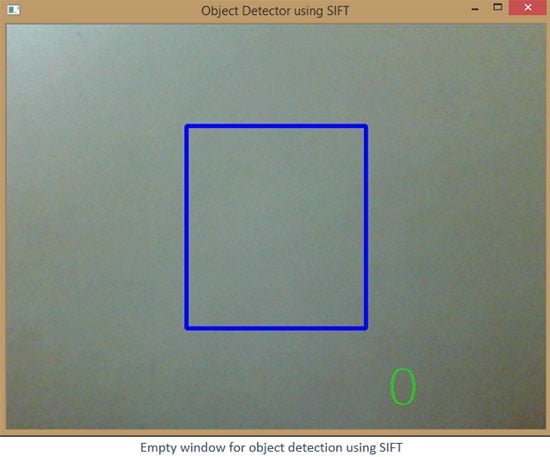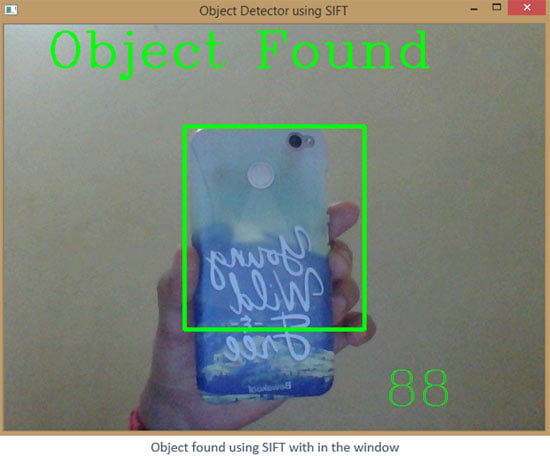### Object detection using ORB

Object detection using SIFT is pretty much cool and accurate, since it generates a much accurate number of matches based on keypoints, however its patented and that makes it hard for using it for the commercial applications, the other way out for that is the ORB algorithm for object detection.

Similar to the method of object detection by SIFT in which we divided the programme into two parts, the same will be followed here.

Firstly, we define the function ORB_detector which takes two inputs one is the live stream image coming from webcam and other is the image template on the basis of which we are going to match our image. Then we grayscale our webcam image and then initialize our ORB detector, and we are setting it here at 1000 key points and scaling parameters of 1.2. you can easily play around with these parameters, then detect the keypoints (kp) and descriptors (des) for both the images and the second parameter we are defining in detectANDCompute function is NONE, it is asking for the use of image mask or not and we are denying it here.

Then move to the detector previously we have been using FLANN based matcher, but here we will be using BFMatcher and inside BFMatcher we define two parameters one is NORM_HAMMING and other is the crossCheck whose value is TRUE.

Then compute the matches the matches between those two images using the descriptors defined above, which in all returns the number of matches since these matches are not approximation and hence there is no need to do Lowe’s ratio test, instead we sort the matches based upon distance, least the distance more the match is better (here the distance means distance between the points), and at the end we return the number of matches using length function.

And in the main function we set the threshold to a much higher value, since orb detector generates much of noise.

Now let’s look at code for ORB based detection

```import cv2
import numpy as np

def ORB_detector(new_image, image_template):
# Function that compares input image to template
# It then returns the number of ORB matches between them
image1 = cv2.cvtColor(new_image, cv2.COLOR_BGR2GRAY)

# Create ORB detector with 1000 keypoints with a scaling pyramid factor of 1.2
orb = cv2.ORB_create(1000, 1.2)

# Detect keypoints of original image
(kp1, des1) = orb.detectAndCompute(image1, None)

# Detect keypoints of rotated image
(kp2, des2) = orb.detectAndCompute(image_template, None)

# Create matcher
# Note we're no longer using Flannbased matching
bf = cv2.BFMatcher(cv2.NORM_HAMMING, crossCheck=True)
# Do matching
matches = bf.match(des1,des2)

# Sort the matches based on distance.  Least distance
# is better
matches = sorted(matches, key=lambda val: val.distance)
return len(matches)

cap = cv2.VideoCapture(0)

# Load our image template, this is our reference image

while True:
# Get webcam images

# Get height and width of webcam frame
height, width = frame.shape[:2]

# Define ROI Box Dimensions (Note some of these things should be outside the loop)
top_left_x = int(width / 3)
top_left_y = int((height / 2) + (height / 4))
bottom_right_x = int((width / 3) * 2)
bottom_right_y = int((height / 2) - (height / 4))

# Draw rectangular window for our region of interest
cv2.rectangle(frame, (top_left_x,top_left_y), (bottom_right_x,bottom_right_y), 255, 3)

# Crop window of observation we defined above
cropped = frame[bottom_right_y:top_left_y , top_left_x:bottom_right_x]

# Flip frame orientation horizontally
frame = cv2.flip(frame,1)

# Get number of ORB matches
matches = ORB_detector(cropped, image_template)

# Display status string showing the current no. of matches
output_string = "Matches = " + str(matches)
cv2.putText(frame, output_string, (50,450), cv2.FONT_HERSHEY_COMPLEX, 2, (250,0,150), 2)

# Our threshold to indicate object deteciton
# For new images or lightening conditions you may need to experiment a bit
# Note: The ORB detector to get the top 1000 matches, 350 is essentially a min 35% match
threshold = 250

# If matches exceed our threshold then object has been detected
if matches > threshold:
cv2.rectangle(frame, (top_left_x,top_left_y), (bottom_right_x,bottom_right_y), (0,255,0), 3)
cv2.putText(frame,'Object Found',(50,50), cv2.FONT_HERSHEY_COMPLEX, 2 ,(0,255,0), 2)

cv2.imshow('Object Detector using ORB', frame)
if cv2.waitKey(1) == 13: #13 is the Enter Key
break

cap.release()
cv2.destroyAllWindows()```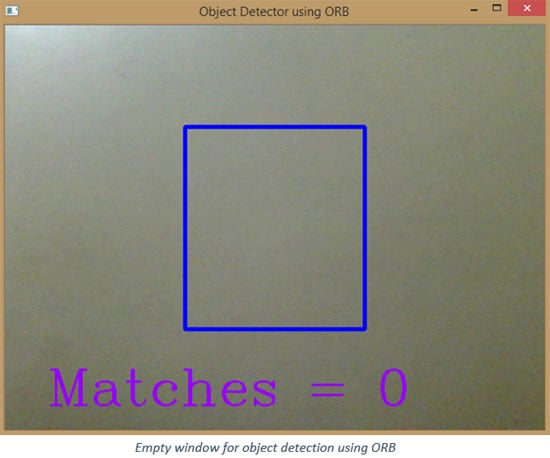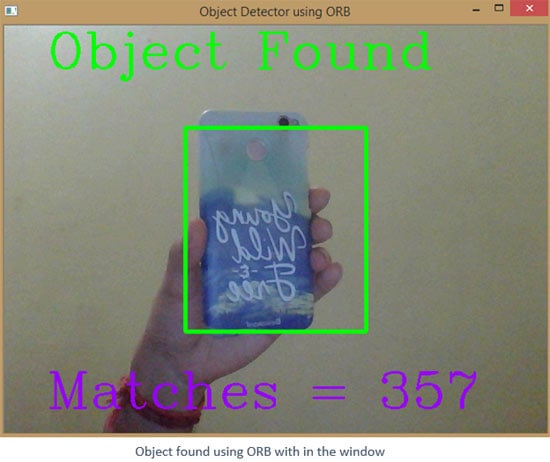### Histogram of Oriented Gradients (HOG’s)

Now let’s talk about a different descriptor which is Histogram of Oriented Gradients (HOG’s).

HOG’s are pretty much cool and useful descriptors and they are widely and successfully used for object detection, as seen previously the image descriptors like SIFT and ORB where we have to compute keypoints and then have to compute descriptors out of those keypoints, HOG’s do that process differently. It represents objects as a single feature vector as opposed to a set of feature vectors where each represents a segment of the image. It means we have single vector feature for the entire image.

It’s computed by a sliding window detector over an image, where a HOG descriptor is a computed for each position. And then each position is combined for a single feature vector.

Like SIFT the scale of the image is adjusted by pyramiding.

Previously we have used matchers like FLANN and BFMatcher, but HOGs do it differently with the help of SVM (support vector machine) classifiers, where each HOG descriptor that is computed is fed to a SVM classifier to determine if the object was found or not.

Here’s the link to a Great Paper by Dalal & Triggs on using HOGs for Human Detection:
https://lear.inrialpes.fr/people/triggs/pubs/Dalal-cvpr05.pdf

### Histogram of Oriented Gradients (HOG’s), Step by Step:

Understanding HOG’s could be quite complex, but here we are only going to deal with the theory of HOG’s without going deeper into the mathematics related to it.

So let’s take this picture it’s a little pixelated a bit, and on the upper corner is 8x8 pixel box here, so in this box we compute the gradient vector or edge orientations at each pixel. So it means in this box we calculate the image gradient vector of pixels inside the box (they are sort of direction or flow of the image intensity itself), and this generates 64 (8 x 8) gradient vectors which are then represented as a histogram. So imagine a histogram which represents each gradient vector. So if all the points or intensities lied in one direction, the histogram for that direction let’s say 45 degrees, the histogram would have peak at 45 degrees.

So what we do now is we split each cell into angular bins, where each bin corresponds to a gradient direction (e.g. x, y). In the Dalal and Triggs paper, they used 9 bins0-180° (20° each bin). This effectively reduces 64 vectors to just 9 values. So what we have done is reduced the size but kept all the key information which is needed.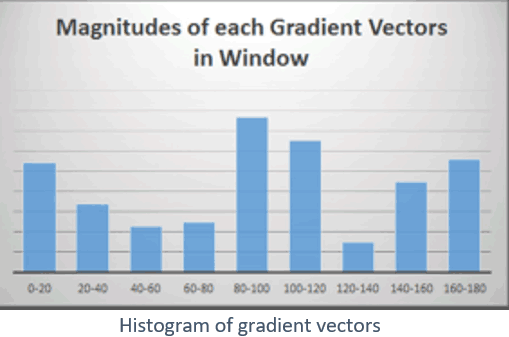Next step in calculating the hog’s is the normalization, we normalize the gradients to ensure invariance to illumination changes i.e. Brightness and Contrast.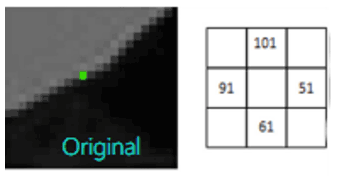In this image, the intensity values are shown in the square according to the respective direction and all have difference of 50 between each other

`∆H = 50, ∆v = 50; │∆│= √502+50 = 70.72, 70.72/100=0.707`

We divide the vectors by the gradient magnitudes we get 0.707 for all, this is normalization.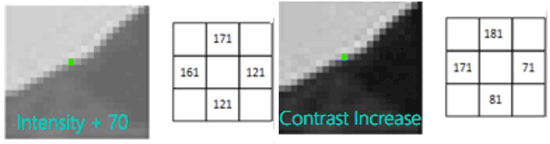Similarly, if we change the intensity or change the contrast we get the below values.

`∆H = 50, ∆v = 50; │∆│= √502+50 = 70.72, 70.72/100=0.707; ∆H = 100, ∆v = 100; │∆│= √1002+100 =141.42, 141.42/100=1.41`

Normalization doesn’t take place on a cell level, instead it takes place in a block level, so here the blocks are basically a group of 4 cells, this takes into account neighboring blocks so normalize while taking into consideration larger segments of the image.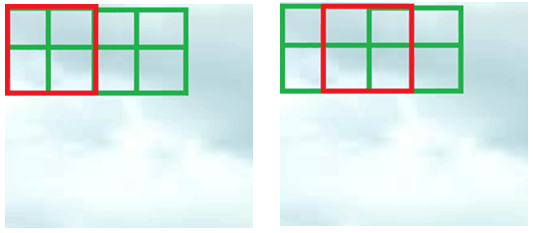Now let’s look at the code

```import numpy as np
import cv2
import matplotlib.pyplot as plt

gray = cv2.cvtColor(image, cv2.COLOR_BGR2GRAY)

# Show original Image
cv2.imshow('Input Image', image)
cv2.waitKey(0)

#defining the parameters, cell size and block size
# h x w in pixels
cell_size = (8, 8)

# h x w in cells
block_size = (2, 2)

# number of orientation bins
nbins = 9

# Using OpenCV's HOG Descriptor
# winSize is the size of the image cropped to a multiple of the cell size
hog = cv2.HOGDescriptor(_winSize=(gray.shape // cell_size * cell_size,
gray.shape // cell_size * cell_size),
_blockSize=(block_size * cell_size,
block_size * cell_size),
_blockStride=(cell_size, cell_size),
_cellSize=(cell_size, cell_size),
_nbins=nbins)

# Create numpy array shape which we use to create hog_features
n_cells = (gray.shape // cell_size, gray.shape // cell_size)

# We index blocks by rows first.
# hog_feats now contains the gradient amplitudes for each direction,
# for each cell of its group for each group. Indexing is by rows then columns.
hog_feats = hog.compute(gray).reshape(n_cells - block_size + 1,
n_cells - block_size + 1,
block_size, block_size, nbins).transpose((1, 0, 2, 3, 4))

# Create our gradients array with nbin dimensions to store gradient orientations

# Create array of dimensions
cell_count = np.full((n_cells, n_cells, 1), 0, dtype=int)

# Block Normalization
for off_y in range(block_size):
for off_x in range(block_size):
gradients[off_y:n_cells - block_size + off_y + 1,
off_x:n_cells - block_size + off_x + 1] += \
hog_feats[:, :, off_y, off_x, :]
cell_count[off_y:n_cells - block_size + off_y + 1,
off_x:n_cells - block_size + off_x + 1] += 1

# Plot HOGs using Matplotlib
# angle is 360 / nbins * direction

color_bins = 5
plt.gca().invert_yaxis()
plt.colorbar()
plt.show()
cv2.destroyAllWindows()```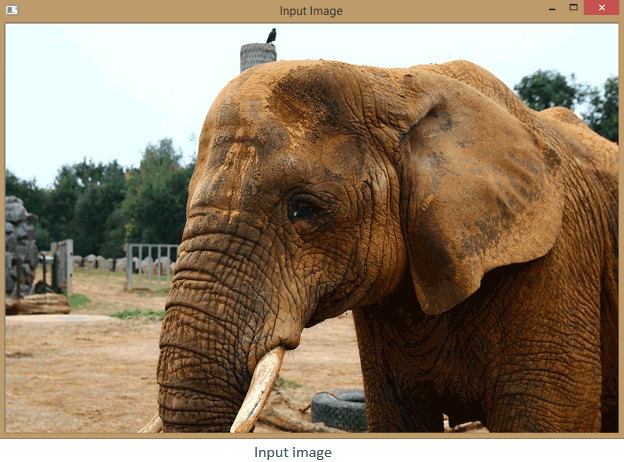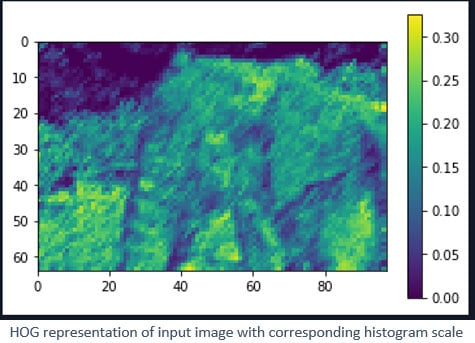The image shows how the input image is represented as HOG representation.

As previously discussed, we can extract features from an image and use those features to classify or detect objects.

An object detection method that inputs Haar features into a series of classifiers (cascade) to identify objects in an image. They are trained to identify one type of object, however, we can use several of them in parallel e.g. detecting eyes and faces together.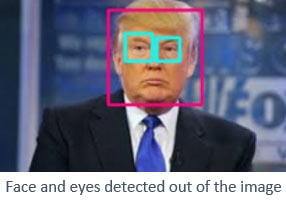HAAR classifiers Explained:

HAAR Classifiers are trained using lots of positive images (i.e. images with the object present) and
negative images (i.e. images without the object present).Once we have those images, we then extract features using sliding windows of rectangular blocks. These features (HAAR features) are single valued and are calculated by subtracting the sum of pixel intensities under the white rectangles from the black rectangles.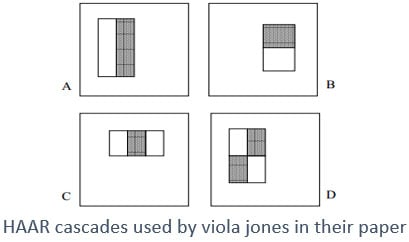However, this is a ridiculous number of calculations, even for a base window of 24 x 24 pixels (180,000 features generated).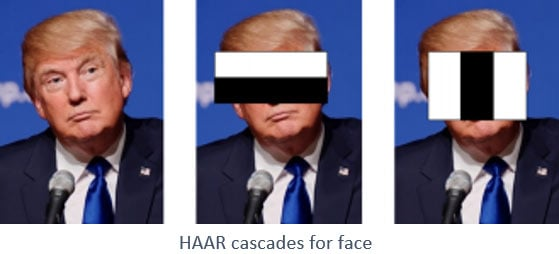So the researchers devised a method called Integral Images that computed this with four array references. However, they still had 180,000 features and the majority of them added no real value.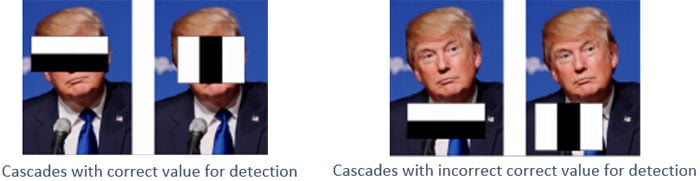Boosting was then used to determine the most informative features, with Freund & Schapire’s AdaBoost and it found most informative features in the image. Boosting is the process by which we use weak classifiers to build strong classifiers, simply by assigning heavier weighted penalties on incorrect classifications. Reducing the 180,000 features to 6000, which is still quite a bit features.

In those 6000 features, some will be more informative than others. So if we used the most informative features to first check whether the region can potentially have a face (false positives will be no big deal). Doing so eliminates the need for calculating all 6000 features at once. This concept is called the Cascade of Classifiers - for face detection, the Viola Jones method used 38 stages.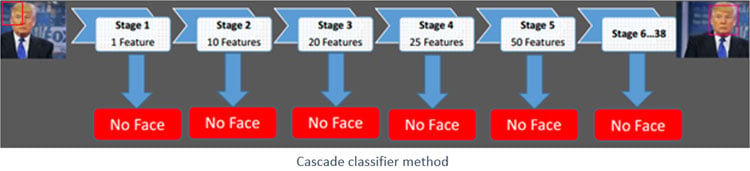### Face & Eye detection

So after gaining some theoretical knowledge about the HAAR cascades we are going to finally implement it, so as to make things pretty much clear we will break the lessons in parts, first we would detect frontal face after that we will move to detect frontal face with eyes and finally we would do live detection of face and eyes through the webcam.

So for this we are going to use pre-trained classifiers that have been provided by OpenCV as .xml files, xml stands for extensible markup language, this language is used to store vast amount of data, you could even build a database on it.

You can have the access of these classifiers at this link.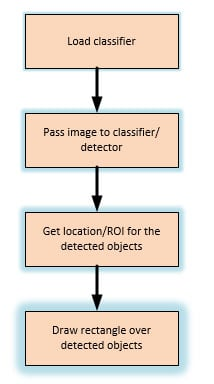Face detection

Let’s try for the frontal face detection, you can have the access for the cascade of frontal face detector here. Just extract the zip file to get the xml file.

```import numpy as np
import cv2

# We point OpenCV's CascadeClassifier function to where our
# classifier (XML file format) is stored, remember to keep the code and classifier in the same folder

# Load our image then convert it to grayscale
gray = cv2.cvtColor(image, cv2.COLOR_BGR2GRAY)

# Our classifier returns the ROI of the detected face as a tuple
# It stores the top left coordinate and the bottom right coordinates
# it returns the list of lists, which are the location of different faces detected.

# When no faces detected, face_classifier returns and empty tuple
if faces is ():
print("No faces found")

# We iterate through our faces array and draw a rectangle
# over each face in faces

for (x,y,w,h) in faces:
cv2.rectangle(image, (x,y), (x+w,y+h), (127,0,255), 2)
cv2.imshow('Face Detection', image)
cv2.waitKey(0)

cv2.destroyAllWindows()```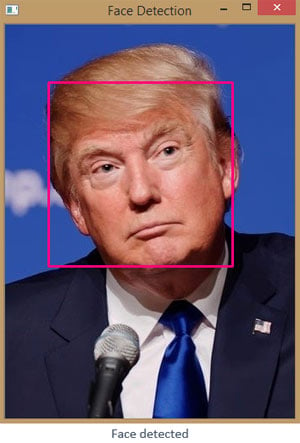Now let’s combine the face and eye detection together, you can have the access for the cascade of eye detector in the same zip file.

```import numpy as np
import cv2

gray = cv2.cvtColor(img, cv2.COLOR_BGR2GRAY)
faces = face_classifier.detectMultiScale(gray, 1.3, 5)

# When no faces detected, face_classifier returns and empty tuple
if faces is ():
print("No Face Found")

for (x,y,w,h) in faces:
cv2.rectangle(img,(x,y),(x+w,y+h),(127,0,255),2)
cv2.imshow('img',img)
roi_gray = gray[y:y+h, x:x+w]
roi_color = img[y:y+h, x:x+w]
eyes = eye_classifier.detectMultiScale(roi_gray)
cv2.waitKey(0)
for (ex,ey,ew,eh) in eyes:
cv2.rectangle(roi_color,(ex,ey),(ex+ew,ey+eh),(255,255,0),2)
cv2.imshow('img',img)
cv2.waitKey(0)

cv2.destroyAllWindows()
cv2.waitKey(0)```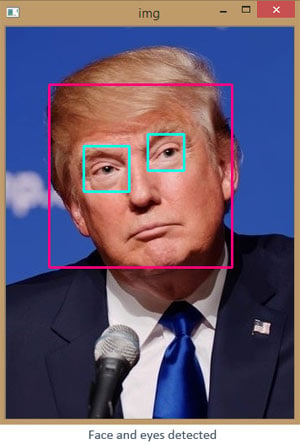So this code is same as much as that the code for the face detection, but here we have added eye cascades and method to detect them, as you can see we have chosen the Gray scaled version of the face as the parameter for the detectMultiScale for the eyes, which brings us to the reduction in computation as we are only going to detect eyes only in that area only.

### Live Face and Eye detection

So till now we have done face and eye detection, now let’s implement the same with the live video stream from the webcam. In this we will do the same detection of face and eyes but this time we will be doing it for the live stream form the webcam. In most of the application you would find your face highlighted with a box around it, but here we have done something differently that you would find your face cropped out and eyes would identify in that only.

So in here we are importing both the face and eye classifier, and defined a function for doing all the processing for the face and eye detection. And after that started the webcam stream and called the face detector function for getting the face and eyes detected. The parameter we are defining inside the face detector function are the continuous images from live web cam stream

```import cv2
import numpy as np

def face_detector(img, size=0.5):
# Convert image to grayscale
gray = cv2.cvtColor(img,cv2.COLOR_BGR2GRAY)
faces = face_classifier.detectMultiScale(gray, 1.3, 5)
if faces is ():
return img

for (x,y,w,h) in faces:
x = x - 50
w = w + 50
y = y - 50
h = h + 50
cv2.rectangle(img,(x,y),(x+w,y+h),(255,0,0),2)
roi_gray = gray[y:y+h, x:x+w]
roi_color = img[y:y+h, x:x+w]
eyes = eye_classifier.detectMultiScale(roi_gray)
for (ex,ey,ew,eh) in eyes:
cv2.rectangle(roi_color,(ex,ey),(ex+ew,ey+eh),(0,0,255),2)

roi_color = cv2.flip(roi_color,1)
return roi_color

cap = cv2.VideoCapture(0)

while True:

cv2.imshow('Our Face Extractor', face_detector(frame))
if cv2.waitKey(1) == 13: #13 is the Enter Key
break

cap.release()
cv2.destroyAllWindows()```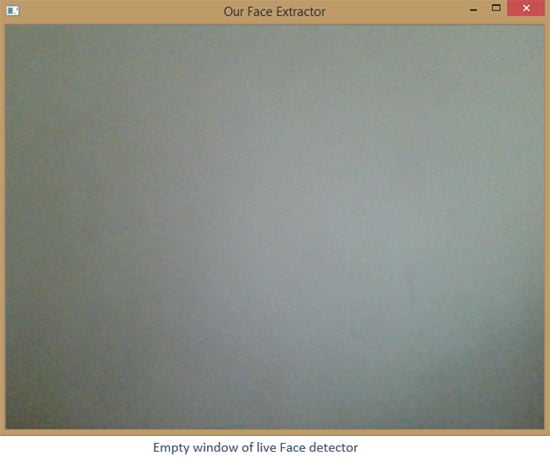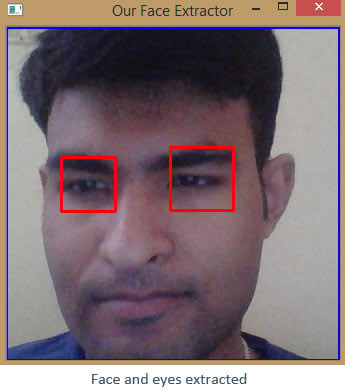The parameters defined inside detectMultiScale other than the input image have the following significance

`ourClassifier.detectMultiScale(input image, Scale Factor , Min Neighbors)`

• Scale Factor Specifies how much we reduce the image size each time we scale. E.g. in face detection we typically use 1.3. This means we reduce the image by 30% each time it’s scaled. Smaller values, like 1.05 will take longer to compute, but will increase the rate of detection.
• Min Neighbors Specifies the number of neighbors each potential window should have in order to consider it a positive detection. Typically set between 3-6. It acts as sensitivity setting, low values will sometimes detect multiples faces over a single face. High values will ensure less false positives, but you may miss some faces.

### Car and Pedestrian Detection in videos

Now we will detect pedestrian and cars in videos using the HAAR cascades, but in the case no video is loading and code compiles without an error you need to follow the following steps:

If no video loads after running code, you may need to copy our opencv_ffmpeg.dl fromopencv\sources\3rdparty\ffmpeg to paste it where your python is installed e.g. C:\Anaconda2

Once it's copied you'll need to rename the file according to the version of OpenCV you're using.e.g. if you're using OpenCV 2.4.13 then rename the file as:opencv_ffmpeg2413_64.dll or opencv_ffmpeg2413.dll (if you're using an X86 machine) opencv_ffmpeg310_64.dll or opencv_ffmpeg310.dll (if you're using an X86 machine)

To find out where you python.exe is installed, just run these two lines of code, it would print the location where python is installed.

```import sys
print(sys.executable)```

Now if you have done these steps successfully, let’s move to the code for pedestrian detection,

You can have the cascade for pedestrian detection and from the zip file attached here.

```import cv2
import numpy as np

# Create our body classifier

# Initiate video capture for video file, here we are using the video file in which pedestrians would be detected
cap = cv2.VideoCapture('walking.avi')

# Loop once video is successfully loaded
while cap.isOpened():

# Reading the each frame of the video

# here we are resizing the frame, to half of its size, we are doing to speed up the classification
# as larger images have lot more windows to slide over, so in overall we reducing the resolution
#of video by half that’s what 0.5 indicate, and we are also using quicker interpolation method that is #interlinear
frame = cv2.resize(frame, None,fx=0.5, fy=0.5, interpolation = cv2.INTER_LINEAR)

gray = cv2.cvtColor(frame, cv2.COLOR_BGR2GRAY)
# Pass frame to our body classifier
bodies = body_classifier.detectMultiScale(gray, 1.2, 3)

# Extract bounding boxes for any bodies identified
for (x,y,w,h) in bodies:
cv2.rectangle(frame, (x, y), (x+w, y+h), (0, 255, 255), 2)
cv2.imshow('Pedestrians', frame)

if cv2.waitKey(1) == 13: #13 is the Enter Key
break

cap.release()
cv2.destroyAllWindows()```

After successfully detecting pedestrian in video, let’s move to the code for Car detection, You can have the cascade for pedestrian detection from here.

```import cv2
import time
import numpy as np

# Create our body classifier

# Initiate video capture for video file
cap = cv2.VideoCapture('cars.avi')

# Loop once video is successfully loaded
while cap.isOpened():
time.sleep(.05)
gray = cv2.cvtColor(frame, cv2.COLOR_BGR2GRAY)

# Pass frame to our car classifier
cars = car_classifier.detectMultiScale(gray, 1.4, 2)

# Extract bounding boxes for any bodies identified
for (x,y,w,h) in cars:

cv2.rectangle(frame, (x, y), (x+w, y+h), (0, 255, 255), 2)
cv2.imshow('Cars', frame)

if cv2.waitKey(1) == 13: #13 is the Enter Key
break

cap.release()
cv2.destroyAllWindows()```

You have noticed that we have added time.sleep(.05), it’s just a delay in frame rate so you can confirm that all the cars are correctly identified, or you can easily remove it just by adding a comment label to it.